Скачать презентацию Drag The trajectory of an ink droplet stream

149bd4259b5bf487a6a9a8e09b3a0ca7.ppt

• Количество слайдов: 9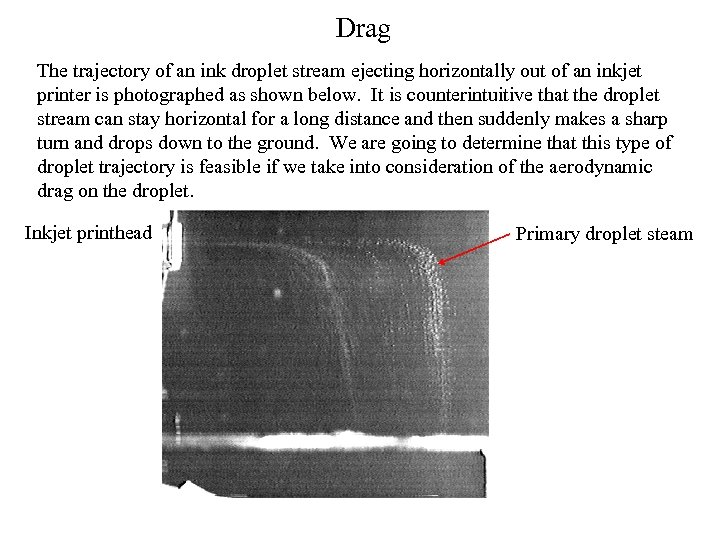Drag The trajectory of an ink droplet stream ejecting horizontally out of an inkjet printer is photographed as shown below. It is counterintuitive that the droplet stream can stay horizontal for a long distance and then suddenly makes a sharp turn and drops down to the ground. We are going to determine that this type of droplet trajectory is feasible if we take into consideration of the aerodynamic drag on the droplet. Inkjet printhead Primary droplet steam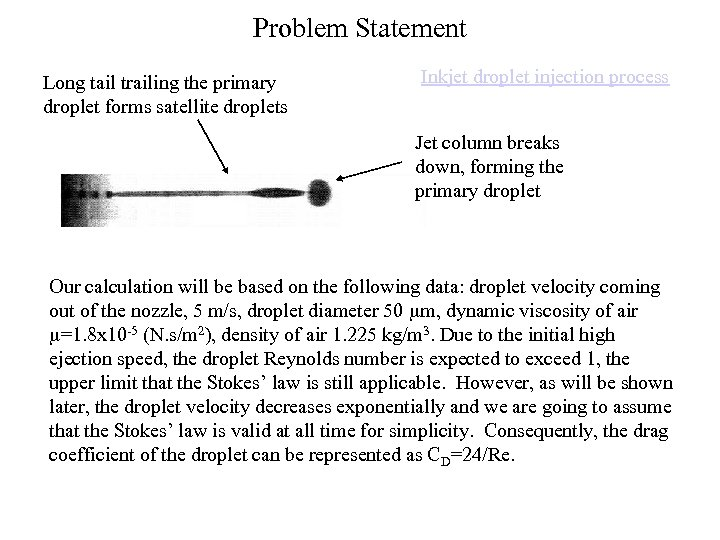Problem Statement Long tail trailing the primary droplet forms satellite droplets Inkjet droplet injection process Jet column breaks down, forming the primary droplet Our calculation will be based on the following data: droplet velocity coming out of the nozzle, 5 m/s, droplet diameter 50 mm, dynamic viscosity of air m=1. 8 x 10 -5 (N. s/m 2), density of air 1. 225 kg/m 3. Due to the initial high ejection speed, the droplet Reynolds number is expected to exceed 1, the upper limit that the Stokes’ law is still applicable. However, as will be shown later, the droplet velocity decreases exponentially and we are going to assume that the Stokes’ law is valid at all time for simplicity. Consequently, the drag coefficient of the droplet can be represented as CD=24/Re.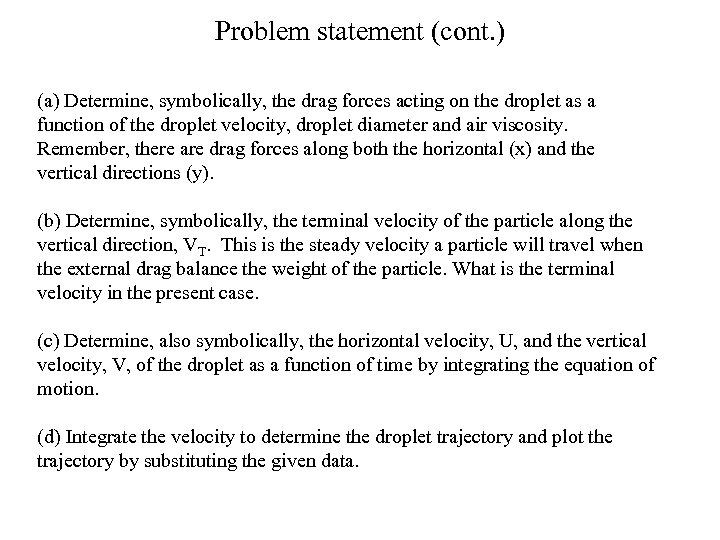Problem statement (cont. ) (a) Determine, symbolically, the drag forces acting on the droplet as a function of the droplet velocity, droplet diameter and air viscosity. Remember, there are drag forces along both the horizontal (x) and the vertical directions (y). (b) Determine, symbolically, the terminal velocity of the particle along the vertical direction, VT. This is the steady velocity a particle will travel when the external drag balance the weight of the particle. What is the terminal velocity in the present case. (c) Determine, also symbolically, the horizontal velocity, U, and the vertical velocity, V, of the droplet as a function of time by integrating the equation of motion. (d) Integrate the velocity to determine the droplet trajectory and plot the trajectory by substituting the given data.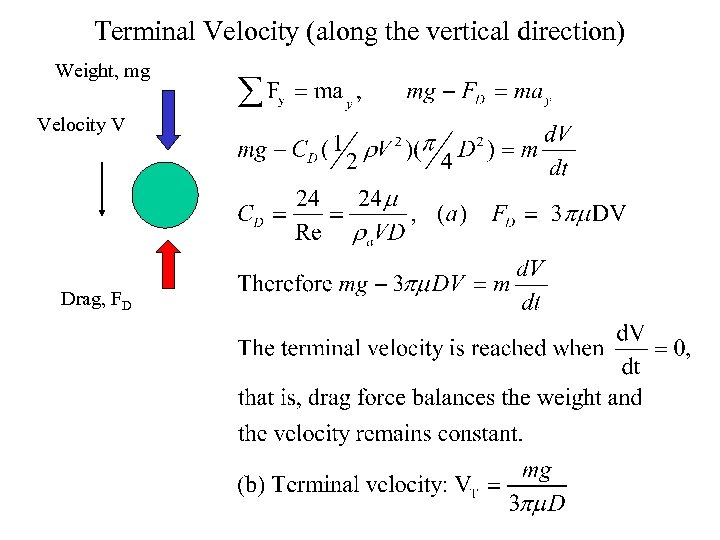Terminal Velocity (along the vertical direction) Weight, mg Velocity V Drag, FD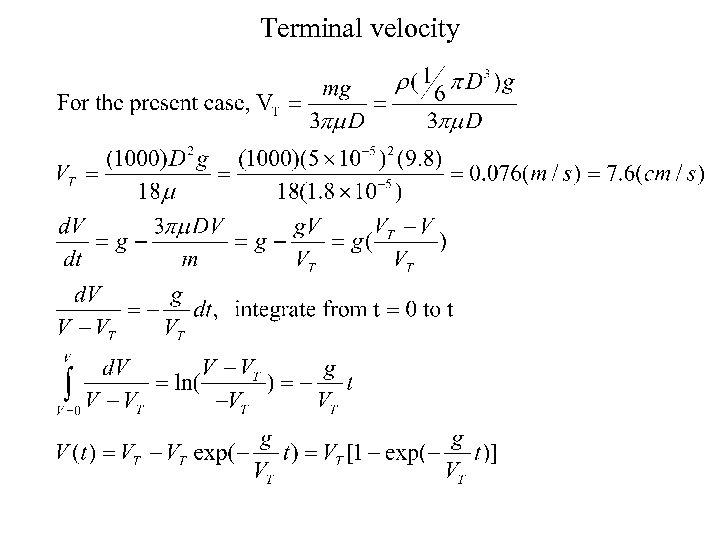Terminal velocity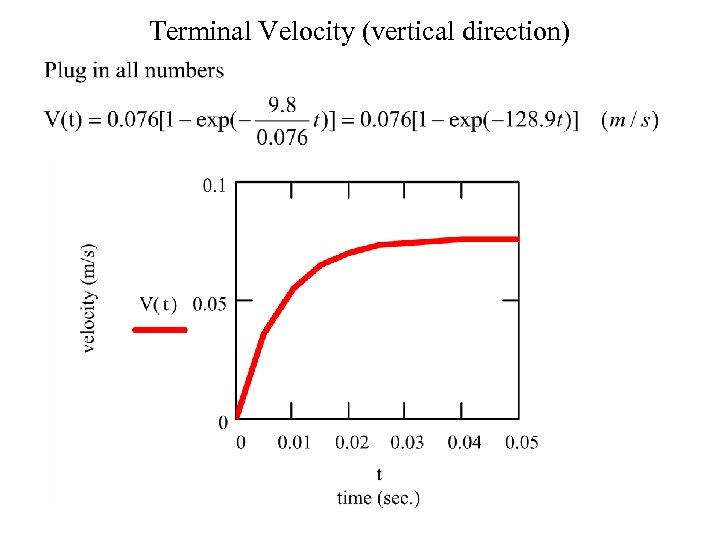Terminal Velocity (vertical direction)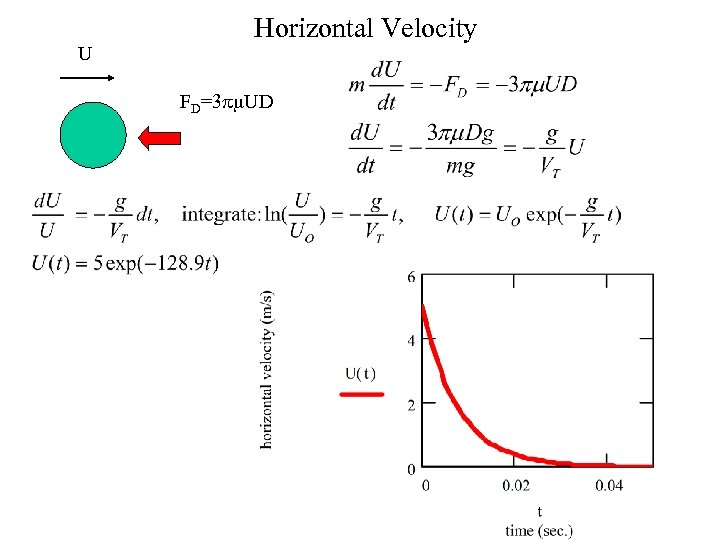U Horizontal Velocity FD=3 pm. UD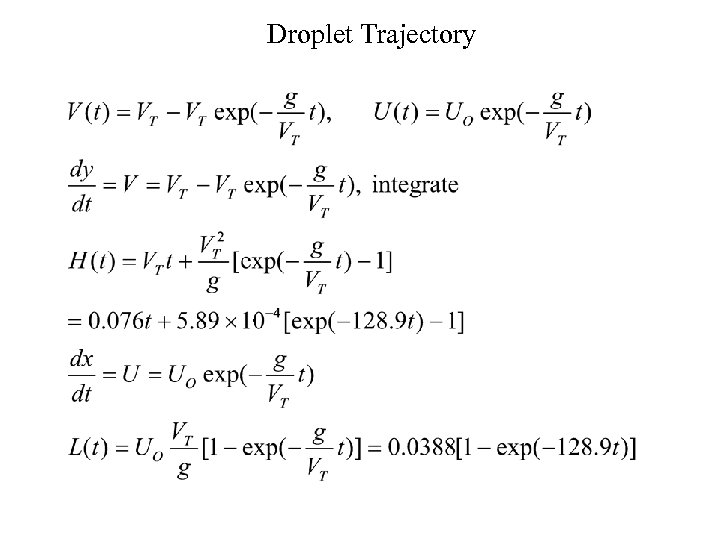Droplet Trajectory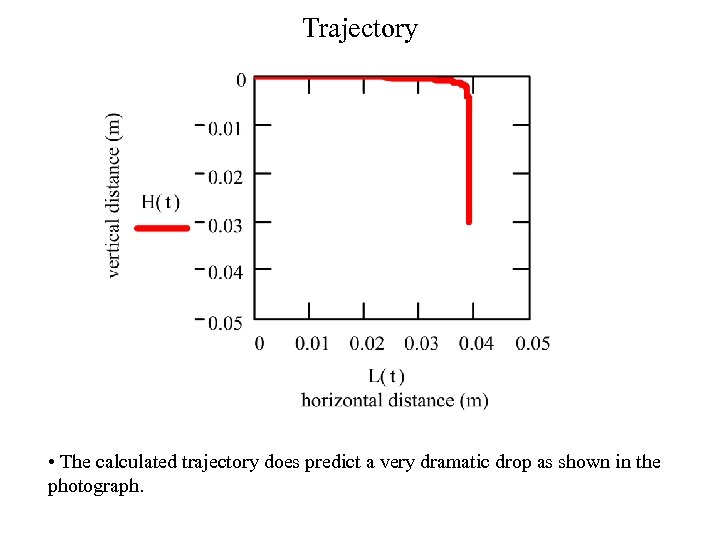Trajectory • The calculated trajectory does predict a very dramatic drop as shown in the photograph.